# Ortak Emiterli Yükselteç / Common Emitter Amplifier

The most common amplifier configuration for NPN transistors is the common emitter/emitter amplifier circuit Introduction to amplifier Circuits, we found that a family of curves, commonly known as output characteristic curves, depended on the transistor collector current (Ic), collector voltage (Vce) for different values of the basic current (Ib) of transistors. Any transistor amplifier works using AC signal inputs ranging from a positive value to a negative value, so a way to "pre-adjust" the amplifier circuit to work between these two maximum or peak values is required. This is achieved using a process known asbiasing. Biasing is very important in the design of the amplifier, since the transistor ready to receive the signals determines the correct working point of the amplifier, thereby reducing the distortion of the output signal. We also found that a static or DC load line can be drawn on the curves of these output properties to show all possible working points of the transistor from completely "on" to completely "off", and the amplifier's silent working point or Q point can be found. The purpose of any small signal amplifier is to raise the entire input signal to the output signal with the minimum amount of distortion possible, that is, the output signal should be a complete reproduction of the input signal, but only larger (reinforced).

## Common Emitter Amplifier CircuitThe single-stage common emitter amplifier circuit shown above uses what is often called "voltage dividing polarization.". This type of polarization (biasing) arrangement uses two resistances as a potential dividing network throughout the feed with the center points that provide the transistor with the necessary basic polarization voltage. Voltage-dividing biasing is widely used in the design of bipolar transistor amplifier circuits.This biasing method of the transistor greatly reduces the effects of the altered Beta (β) by keeping the Base front polarity at the constant voltage level, which allows for optimal stability. Sedentary Base voltage (Etc.), as shown by the current passing through both resistors, the two resistance, R1, R2 and power supply voltage are determined by the potential dividing network generated by the VCC. Then the total resistance RT will be equal to R1 + R2, which gives the current as i = Vcc/RT. The voltage level produced in the combination of R1 and R2 resistors keeps the basic voltage (Etc.) constant at a value below the feed voltage. The potential dividing network, which is then used in the common emitter amplifier circuit, divides the supply voltage proportionally to resistance. This polarising reference voltage can be easily calculated using the simple voltage divider formula below.

## Biasing VoltageThe same supply voltage (Vcc) also determines the maximum collector current, Ic, when the transistor is completely in the "ON" (saturation) position, Vce = 0.

## Beta β Value

Beta is sometimes referred to as hFE, which is the advanced current gain of transistors in the common emitter configuration. Beta two currents have no units because Ic and Ib have a constant ratio, so a small change in base current causes a major change in the collector's current. One last reminder about beta. Transistors with the same type and part number have big differences in Beta values. For example, the BC107 NPN Bipolar transistor has a Beta value between 110 and 450. So one BC107 has a Beta value of 110, while the other may have a Beta value of 450, but both are BC107 NPN transistors. This is because it is a feature of the transistor structure, not the operation of beta. Since the base/emitter connection is forward,there will be a decrease in transmitter voltage, and base voltage. If the voltage on the emitter resistance is known, the transmitter current can be easily calculated using the Ohm Act. Collector current can be approximately calculated as Ic is almost the same value as the emitter current.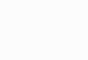## Common Transmitter Amplifier Example

A common emitter amplifier circuit has load resistance, 1.2kΩ RL and 12V supply voltage. When the transistor is completely positioned in the "ON" (saturation) position, calculate the maximum Collector current (Ic) passing through the load resistance, accept Vce = 0.Also, find the RE value of the transmitter resistance when a voltage drop of 1V occurs. Calculate the values of all circuit resistors.This then forms the "A" point on the vertical axis of the collector current of its characteristic curves and occurs when Vce = 0. When the transistor is completely "OFF", there is no voltage drop in RE or RL resistance as no current flows. Then the voltage drop along the transistor is equal to Vce, feed voltage, Vcc. This creates the point "B" on the horizontal axis of its characteristic curves. In general, the sedentary Q point of the amplifier is connected to the zero input signal applied to the Base, so the Collector is fixed at approximately half the level along the load line between zero volt and feed voltage (Vcc/2). Therefore, the Collector current at the amplifier's Q point will be calculated as follows:This static DC load line produces a straight line equation, which is given as -1/(RL + RE) and cuts the vertical Ic axis at a point equal to VCC/(RL + RE). The actual position of the Q point on the DC load line is determined by the average value of the Ib. As collector current, the Ic of the transistor is also equal to the DC gain (Beta) of the transistor, if we assume a Beta (β) value of 100 for the transistor, equal to the Base stream (β*Ib) product. The basic current flowing into the transistor Ib will be calculated as follows:The current flowing from the voltage dividing circuit must be large compared to the actual Base current (Ib), so that the voltage dividing network is not loaded by the Base current flow. A general rule is that the current flowing from the R2 resistance is at least 10 times the value of Ib. The transistor Base/Emitter voltage is fixed at Vbe 0.7V (silicon transistor), in which case the R2 value is calculated as follows:If the current flowing from the R2 resistance is 10 times the value of the base current, the current flowing from the R1 resistance in the dividing network should be 11 times the value of the base current. So: IR2 + Ib.

Thus, the voltage on the R1 resistance equals Vcc – 1.7v (VRE + 0.7 for silicon transistor), which equates to 10.3V, so R1 can be calculated as follows:The value of transmitter resistance can be easily calculated using RE, Ohm Law. The current flowing through RE is a combination of Base current, Ib and Collector current Ic and is calculated as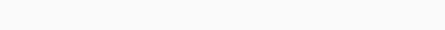follows: Resistance is connected between the emiter terminal of the RE transistors and the soil, and we have previously said that there is a voltage drop of 1 volt on it. Thus, the value of the transmitter resistance, RE, is calculated as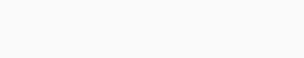follows: Therefore, for our example above, the preferred values of the resistors selected to give a tolerance of 5% are as follows: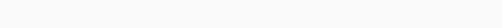Then, our original Common Emitter Amplifier circuit above can be rewritten to include the values of the components we calculated above.## Amplifier Caplin Capacitors

In common Emitter Amplifier circuits, C1 and C2 capacitors are used as Coupling Capacitors to separate AC signals from DC biasing voltage. This ensures that the biasing condition set for the correct operation of the circuit is not affected by any additional amplifier stages, as capacitors will only pass AC signals and block any DC components. The output AC signal is then overlayed on the polarity of the following stages. It is also connected to a bypass capacitor, CE, transmitter leg circuit. This capacitor is an active open circuit component for DC polarity conditions. However, this parallel connected bypass capacitor effectively shorts the transmitter resistance in high frequency signals due to its reactance. Therefore, only RL plus a very small internal resistance acts, maximizing the increased voltage gain in the transistor. In general, the value of the bypass capacitor CE is selected to provide a reassurance of no more than 1/10 of the RE value at the lowest operating signal frequency.

## Characteristic Curves

Now, for our simple common emitter amplifier circuit, we can create a series of curves that show the Collector current, IC against the Collector/Transmitter voltage, showing Vce with different Base current, Ib values. These curves are known as "Output Characteristic Curves" and are used to show how the transistor will work on its dynamic range. A static or DC load line is drawn on curves for a load resistance RL of 1.2kΩ to indicate possible working points for all transistors. When the transistor is positioned in the "OFF" position, Vce is equal to the Vcc supply voltage, which is the "B" point on the line. Likewise, when the transistor is completely "ON" and saturated, the collector current load resistance is determined by the RL and is the "A" point on this line. We have previously calculated from the DC gain of the transistor that the base current required for the average position of the transistor is 45.8μA, which we have marked as the Q point on the load line representing the silent point of the amplifier or the Q point. We can make life quite easy for ourselves and complete this value to exactly 50μA without any effect on the point of work.Point Q on the load line gives us the Base current Q-point of Ib = 45.8μA or 46μA. Without any distortion of the output signal, we need to find the maximum and minimum peak oscillations of the base current, which will result in a proportional change in the collector's current, IC. When cutting different Base current values on the load line, DC characteristic curves, we can find peak oscillations of the Base current, which are evenly spaced along the load line. These values are marked as "N" and "M" points on the line and give a minimum and maximum Base current of 20μA and 80μA respectively. These points can be anywhere along the freight line we choose, as long as "N" and "M" are evenly spaced from Q. This gives us a theoretical maximum entry signal to the base terminal from 60μA top to top.Any input signal that gives a base current greater than this value removes the transistor beyond the "N" point and beyond the "interrupt" zone or "M" point and out of the saturation zone, thereby causing the output signal to deteriorate. For example, using the "N" and "M" points, the instantaneous values of the collector stream and the corresponding values of the Collector-emitter voltage can be reflected from the load line. Collector-emitter voltage can be seen to be antiphased (–180o) with collector current. When the base current changes from 50μA to 80μA in a positive direction, the collector-emitter voltage, which is also the output voltage, decreases from 5.8 volts to 2.0 volts. Then the single-stage Common Emitter Amplifier is also an "Inverter amplifier", since an increase in base voltage causes a decrease in Vout, and a decrease in base voltage causes an increase in Vout. In other words, the output signal is out of phase 180o with the input signal.

## Common Transmitter Voltage Gain

The Voltage Gain of the common emitter amplifier is equal to the ratio of the change in input voltage to the change in the output voltage of the amplifier. Then it is ΔVL = Vout and ΔVB = Vin. However, the voltage gain is also equal to the ratio of signal resistance in the Collector to signal resistance in the Transmitter and is calculated as follows: As the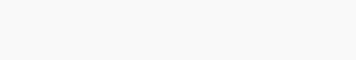signal frequency increases the bypass capacitor, CE begins to short-circuit transmitter resistance due to its recess. Then, at high frequencies, RE = 0 makes the gain infinite. However, bipolar transistors have a small internal resistance placed in donor regions called Re. The semiconductor material of transistors offers an internal resistance to the current passing through it and is usually represented by a small symbol of resistance, which is shown inside the main transistor symbol. Transistor data pages tell us that for a small signal bipolar transistors, this internal resistance is the product of 25mV ÷ Ie (25mV is the internal voltage drop along the Transmitter connection layer), then the value above this resistance value for our common Transmitter amplifier circuit will be equal. For: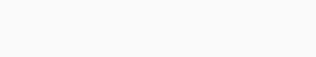This built-in Transmitter leg resistance will be serialized with external Transmitter resistance RE, then the equation of the actual gain of transistors will be changed to include this internal resistance, and it will be as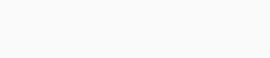follows: In low frequency signals, the total resistance in the Transmitter leg is equal to RE + Re. At high frequency, the bypass capacitor shorts the Transmitter resistor, leaving only the internal resistance Re in the Transmitter leg to provide high gain. Then, for our common emitter amplifier circuit above, the gain of the circuit at both low and high signal frequencies is given as follows:

### Gain at Low Frequencies### Gain at High Frequencies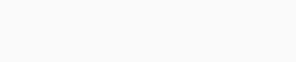One last point, voltage gain depends only on collector resistance, RL and Transmitter resistance values, (RE + Re) the current gain of the transistor is not affected by Beta, β (hFE). That is, for our simple example above we can now summarize all the values that we have calculated for our common emitter amplifier circuit, and these are:

 Minimum Plaza Maximum Base Current 20μA 50μA 80μA Collector Stream 2.0mA 4.8mA 7.7mA Output Voltage 2.0V 5.8V 9.3V Riser Gain -5.32 -218

## Summary

The Base of the transistor used in a common emitter amplifier is polarated using two resistors as a potential dividing network. This type of polarizing arrangement is widely used in the design of bipolar transistor amplifier circuits and greatly reduces the effects of beta (β), which changes by keeping base polarity at a constant voltage. This type of polaration produces the greatest stability.

A resistance can be included in the transmitter leg, in which case the voltage gain is -RL/RE. If there is no External Transmitter resistance, the voltage gain of the amplifier is not infinite, as the transmitter has a very small internal resistance in the leg. The value of this internal resistance is equal to 25mV/IE.

In the next lesson on transistor amplifiers, we will look at the Junction Area Effect Amplifier, commonly called the JFET Amplifier. Like the transistor, JFET is used in a single-stage amplifier circuit, making it easy to understand. There are several different types of field-effective transistors that we can use, but the easiest to understand is the effective transistor that receives connectivity or JFET, which has a very high input impedance and makes it ideal for amplifier circuits.